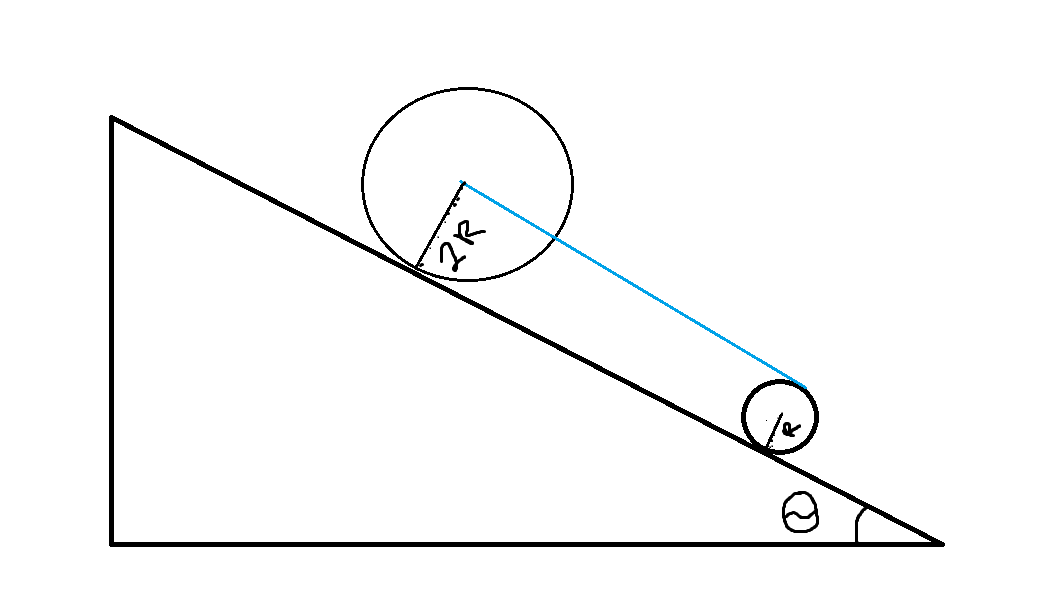# Will you roll with me?Two rigid uniform cylinders each of mass $50kg$ are placed on a rigid and rough inclined plane at an angle of $30^{\circ}$ above the horizontal. A massless string connects the axle of the larger cylinder to the rim of the smaller one. If both of the cylinders roll without slipping, what is the acceleration of the smaller cylinder?

Details and Assumptions

$g$ is $9.81\frac{m}{s^2}$

×